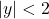The Graduate Record Exam, GRE, includes an analytical section that tests your math skills. One type of question they ask is on inequalities.

In this blog post, we share with you an example of the type of question on inequalities that you can expect to see on the GRE (Graduate Record Exam). The following question is an example of how to solve an inequality:

Question: If, then which all of the following is true?

a)b)c)Approach: First, We need to figure out the range in which y could lie. One short trick will be helpful here. WheneverBased on this, we can easily get the range in which the values of y would lie.

Another important concept is: If a number of raised to a positive integral power, its values increases if it is more than 1, while it decreases if it is between 0 and 1.